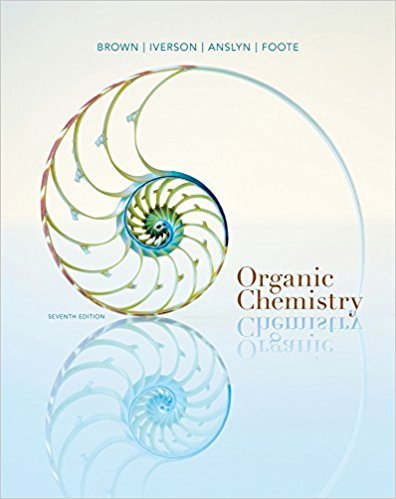×
Get Full Access to Organic Chemistry - 7 Edition - Chapter 4 - Problem 4.36
Get Full Access to Organic Chemistry - 7 Edition - Chapter 4 - Problem 4.36

×

# Write equations for the reaction of each compound with H2SO4, a strong protic acidISBN: 9781133952848 483

## Solution for problem 4.36 Chapter 4

Organic Chemistry | 7th Edition

• Textbook Solutions
• 2901 Step-by-step solutions solved by professors and subject experts
• Get 24/7 help from StudySoup virtual teaching assistantsOrganic Chemistry | 7th Edition

4 5 1 347 Reviews
30
1
Problem 4.36

Write equations for the reaction of each compound with H2SO4, a strong protic acid.

Step-by-Step Solution:
Step 1 of 3

Molecular Nitrogen The contributions to the MO from each of the atoms is unequal – ψ = cAφ(A) + c B(B) +.......The more electronegative atom contributes strongly to the bonding orbital The less electronegative atom contributes strongly to the anti-bonding orbital – gives rise to polarity Orbital mixing The difference in energy between orbitals on different atoms leads to reduced mixing – The reduced mixing does not imply weaker bonding Heteronuclear Molecules The relative energy of the bonding orbitals determines the magnitude of the covalent bond energy (ΔE cov) Hydrogen Fluoride In H-F the 1s orbital of H is energetically well above the 1s and 2s orbitals of F – it interacts only with the 2z orbital (all remaining electrons are in non- bonding orbitals!) Lithium Fluoride As the polarity difference between two atoms increases, the orbital energy difference also increases – electrons shift towards the more electronegative atom Limiting case: Ionic compounds

Step 2 of 3

Step 3 of 3

##### ISBN: 9781133952848

The full step-by-step solution to problem: 4.36 from chapter: 4 was answered by , our top Chemistry solution expert on 03/16/18, 05:02PM. Since the solution to 4.36 from 4 chapter was answered, more than 245 students have viewed the full step-by-step answer. This full solution covers the following key subjects: . This expansive textbook survival guide covers 24 chapters, and 1131 solutions. This textbook survival guide was created for the textbook: Organic Chemistry, edition: 7. The answer to “Write equations for the reaction of each compound with H2SO4, a strong protic acid.” is broken down into a number of easy to follow steps, and 14 words. Organic Chemistry was written by and is associated to the ISBN: 9781133952848.

Unlock Textbook Solution